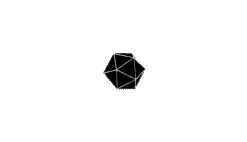# 结构

ControlNet是一种网络结构，可以增强特定任务下的预训练模型。ControlNet对神经网络块中的输入条件进行操作从而控制整个神经网络，这里网络块指的是resnet块、CBN块、多头注意力块、transformer块等常用于搭建神经网络的层。其结构如下图所示。ControlNet锁住原始网络的参数$\Theta$，并且拷贝了一份可以训练的参数$\Theta_c$，除此以外还有额外的条件向量$c$。采用拷贝而不是直接训练原始权重是为了避免数据集过小的时候过拟合并且保留从数十亿张图像中训练到的大型模型的质量。

convolution）进行连接，零卷积即1*1大小的卷积，其权重和偏差都被初始化为0。这么设置使得一开始即使ControlNet不进行训练，ControlNet部分的输入和输出都为0加到原始网络里面也不会对原始网络有任何影响。公式推导如下：

$y=F(x;\Theta)$

$y_c = F(x;\Theta) + Z(F(x+Z(c;\theta_{z_1});\theta_c);\theta_{z_2})$

$\begin{cases} Z(c;\theta_{z_1}) = 0 \\ F(x+Z(c;\theta_{z_1});\theta_c) = F(x;\theta_c) = F(x;\theta) \\ Z(F(x+Z(c;\theta_{z_1});\theta_c);\theta_{z_2}) = Z(F(x;\theta_c);\theta_{z_2}) = 0 \end{cases}$

## 零卷积是怎么学习的

$y = wx + b$

$\frac{\partial y}{\partial w} = x \frac{\partial y}{\partial x} = w \frac{\partial y}{\partial b} = 1$

$w=0 x \ne 0$时，梯度如下

$\frac{\partial y}{\partial w} \ne 0 \frac{\partial y}{\partial x} = 0 \frac{\partial y}{\partial b} \ne 0$

# 整体结构# 参考文献

Adding Conditional Control to Text-to-Image Diffusion Models

ControlNet